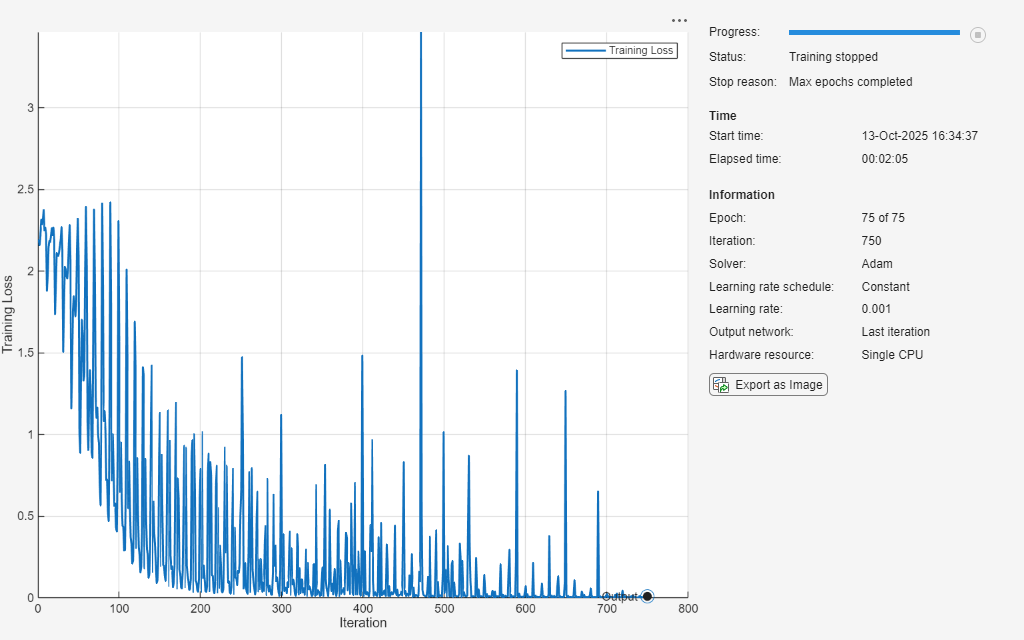## Train Network Using Out-of-Memory Sequence Data

This example shows how to train a deep learning network on out-of-memory sequence data by transforming and combining datastores.

A transformed datastore transforms or processes data read from an underlying datastore You can use a transformed datastore as a source of training, validation, test, and prediction data sets for deep learning applications. Use transformed datastores to read out-of-memory data or to perform specific preprocessing operations when reading batches of data. When you have separate datastores containing predictors and labels, you can combine them so you can input the data into a deep learning network.

When training the network, the software creates mini-batches of sequences of the same length by padding, truncating, or splitting the input data. For in-memory data, the `trainingOptions` function provides options to pad and truncate input sequences, however, for out-of-memory data, you must pad and truncate the sequences manually.

Load the Japanese Vowels data set as described in  and . The zip file `japaneseVowels.zip` contains sequences of varying length. The sequences are divided into two folders, `Train` and `Test`, which contain training sequences and test sequences, respectively. In each of these folders, the sequences are divided into subfolders, which are numbered from `1` to `9`. The names of these subfolders are the label names. A MAT file represents each sequence. Each sequence is a matrix with 12 rows, with one row for each feature, and a varying number of columns, with one column for each time step. The number of rows is the sequence dimension and the number of columns is the sequence length.

Unzip the sequence data.

```filename = "japaneseVowels.zip"; outputFolder = fullfile(tempdir,"japaneseVowels"); unzip(filename,outputFolder);```

For the training predictors, create a file datastore and specify the read function to be the `load` function. The `load` function, loads the data from the MAT-file into a structure array. To read files from the subfolders in the training folder, set the `'IncludeSubfolders'` option to `true`.

```folderTrain = fullfile(outputFolder,"Train"); fdsPredictorTrain = fileDatastore(folderTrain, ... 'ReadFcn',@load, ... 'IncludeSubfolders',true);```

Preview the datastore. The returned struct contains a single sequence from the first file.

`preview(fdsPredictorTrain)`
```ans = struct with fields: X: [12×20 double] ```

For the labels, create a file datastore and specify the read function to be the `readLabel` function, defined at the end of the example. The `readLabel` function extracts the label from the subfolder name.

```classNames = string(1:9); fdsLabelTrain = fileDatastore(folderTrain, ... 'ReadFcn',@(filename) readLabel(filename,classNames), ... 'IncludeSubfolders',true);```

Preview the datastore. The output corresponds to the label of the first file.

`preview(fdsLabelTrain)`
```ans = categorical 1 ```

### Transform and Combine Datastores

To input the sequence data from the datastore of predictors to a deep learning network, the mini-batches of the sequences must have the same length. Transform the datastore using the `padSequence` function, defined at the end of the datastore, that pads or truncates the sequences to have length 20.

```sequenceLength = 20; tdsTrain = transform(fdsPredictorTrain,@(data) padSequence(data,sequenceLength));```

Preview the transformed datastore. The output corresponds to the padded sequence from the first file.

`X = preview(tdsTrain)`
```X = 1×1 cell array {12×20 double} ```

To input both the predictors and labels from both datastores into a deep learning network, combine them using the `combine` function.

`cdsTrain = combine(tdsTrain,fdsLabelTrain);`

Preview the combined datastore. The datastore returns a 1-by-2 cell array. The first element corresponds to the predictors. The second element corresponds to the label.

`preview(cdsTrain)`
```ans = 1×2 cell array {12×20 double} {} ```

### Define LSTM Network Architecture

Define the LSTM network architecture. Specify the number of features of the input data as the input size. Specify an LSTM layer with 100 hidden units and to output the last element of the sequence. Finally, specify a fully connected layer with output size equal to the number of classes, followed by a softmax layer and a classification layer.

```numFeatures = 12; numClasses = numel(classNames); numHiddenUnits = 100; layers = [ ... sequenceInputLayer(numFeatures) lstmLayer(numHiddenUnits,'OutputMode','last') fullyConnectedLayer(numClasses) softmaxLayer classificationLayer];```

Specify the training options. Set the solver to `'adam'` and `'GradientThreshold'` to 2. Set the mini-batch size to 27 and set the maximum number of epochs to 75. The datastores do not support shuffling, so set `'Shuffle'` to `'never'`.

Because the mini-batches are small with short sequences, the CPU is better suited for training. Set `'ExecutionEnvironment'` to `'cpu'`. To train on a GPU, if available, set `'ExecutionEnvironment'` to `'auto'` (the default value).

```miniBatchSize = 27; options = trainingOptions('adam', ... 'ExecutionEnvironment','cpu', ... 'MaxEpochs',75, ... 'MiniBatchSize',miniBatchSize, ... 'GradientThreshold',2, ... 'Shuffle','never',... 'Verbose',0, ... 'Plots','training-progress');```

Train the LSTM network with the specified training options.

`net = trainNetwork(cdsTrain,layers,options);`### Test the Network

Create a transformed datastore containing the held-out test data using the same steps as for the training data.

```folderTest = fullfile(outputFolder,"Test"); fdsPredictorTest = fileDatastore(folderTest, ... 'ReadFcn',@load, ... 'IncludeSubfolders',true); tdsTest = transform(fdsPredictorTest,@(data) padSequence(data,sequenceLength));```

Make predictions on the test data using the trained network.

`YPred = classify(net,tdsTest,'MiniBatchSize',miniBatchSize);`

Calculate the classification accuracy on the test data. To get the labels of the test set, create a file datastore with the read function `readLabel` and specify to include subfolders. Specify that the outputs are vertically concatenateable by setting the `'UniformRead'` option to `true`.

```fdsLabelTest = fileDatastore(folderTest, ... 'ReadFcn',@(filename) readLabel(filename,classNames), ... 'IncludeSubfolders',true, ... 'UniformRead',true); YTest = readall(fdsLabelTest);```
`accuracy = mean(YPred == YTest)`
```accuracy = 0.9351 ```

### Functions

The `readLabel` function extracts the label from the specified filename over the categories in `classNames`.

```function label = readLabel(filename,classNames) filepath = fileparts(filename); [~,label] = fileparts(filepath); label = categorical(string(label),classNames); end```

The `padSequence` function pads or truncates the sequence in `data.X` to have the specified sequence length and returns the result in a 1-by-1 cell.

```function sequence = padSequence(data,sequenceLength) sequence = data.X; [C,S] = size(sequence); if S < sequenceLength padding = zeros(C,sequenceLength-S); sequence = [sequence padding]; else sequence = sequence(:,1:sequenceLength); end sequence = {sequence}; end```